Now we can transfer any of these characters to your own WoW Account.

Name Change Service HERE!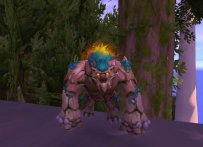Druid = 468 ilvl = 2500 RIO SCORE = 3/5 Set S3 = Mage Tower Guardian = CM GOLD WOD ...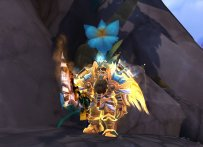PALADIN = 465 ilvl = 4/5 Tier Set S3 = 2.3K RIO SCORE S3 = Mage Tower = THUNDERFURY =...Demon Hunter 460 ilvl = 2/5 Tier Set S3 = Bis Trinket 483 ilvl = 2.2K SCORE = THUNDER...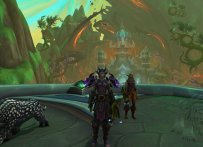Hunter BM = 460 ilvl = 4/5 Tier set S3 = 2200 RIO SCORE S3 = CM GOLD WOD = FLY SL/BFA/...Priest = 453 ilvl = 2/5 Tier Set S3 = Bis Trinkets = Mage Tower = CM GOLD WOD = FLY S...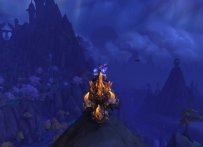MAGE = 438 ilvl = ARENA MASTER =4/5 Tier Set = 2450 RIO SCORE Season 2 = Mage Tower = A...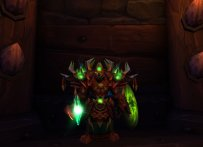Shaman = 456 ilvl = 3/5 Tier Set S3 = 2.1K RIO SCORE S3 = Mage Tower = CM GOLD WOD = ...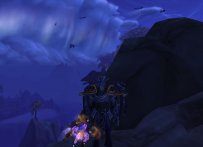Evoker 457 ilvl = 3/5 Tier Set S3 = 2K RIO SCORE = CM GOLD WOD = FLY SH/BFA/LEGION/WOD...Warlock = 450 ilvl = Mage Tower =2/5 Tier Set S3= Bis Trinket 476ilvl = CM GOLD WOD =...INSANE = 25.040 Achievements = S15 Gladiator = Tier 3 = MOP & WOD CM = Elite Legion...Rogue = 450 ilvl = 1/5 Tier Set S3 = Bis Trinket 483 = Mage Tower = Thunderfury = CM...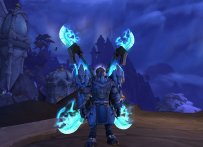Warrior = 450 ilvl = 1/5 Tier Set S3 = Mage Tower = Thunderfury = CM GOLD WOD = FLY SL...Monk WW = 448 ilvl = Mage Tower = 2/5 Tier Set S3 = THUNDERFURY = Weapon 476 ilvl = ...12175 Achievs Points = 6x 70 / 26x 60+ Chars = Notable mounts and Transmogs = 650 EUR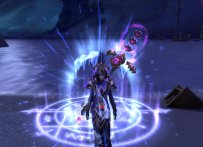Mage = 424 ilvl = 2/5 Tier Set Season 2 = Mage Tower = CM GOLD WOD = FLY SL/BFA/LEGION/...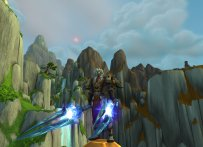DK 390 ilvl = TUSKS OF MANNOROTH = MAGE TOWER = THUNDERFURY = CM GOLD WOD = FLY BFA/LE...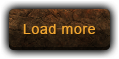X